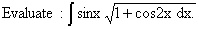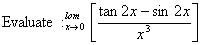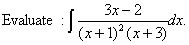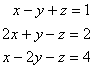Wednesday 14th April 2021

CBSE Guess > Papers > Question Papers > Class XII > 2006 > Mathematics > Compartment Delhi Set - II.

Mathematics — 2006 (Set II — Compartment Delhi)

SECTION - A

Q. 1. In a box containing 50 bulbs 5 are defective. What is the probability that a sample of 5 bulbs will have at most 2 defective bulbs?

Q. 2.Q. 9.Q. 12.Q. 14. The two equal sides of an isosceles tri angle with fixed base b cm are decreasing at the rate of 3 cm/sec. How fast is the area decreasing when the two equal sides are equal to the base?

Q. 17. Using matrices, solve the following system of linear equations:SECTION - B

Q. 19. Using vectors, prove that the perpendicular bisectors of the sides of a triangle are con current.

Q. 21. Driving a train at the speed of 72 km/ .hr a driver noted that he has to stop the train 10 m before the signal which was just 110 m away. Find the retardation and the time taken to stop.

SECTION - C

Q. 20. 2% of the bolts manufactured by a factory are found to be defective. Find the probability that in a packet of 200 bolts not more than 3 bots will come out to be defective.

Q. 22. A bill of exchange drawn on January 4, 2005 at S months was discounted on March 26, 2005 at 3% per annum. If the banker’s discount be Rs. 603.60, find the face value of the bill.

Q. 25. A man borrows Rs. 40,000 at 16% per annum and promises to pay off the loan in 20 annual instalments, beginning at the end of first year. Calculate what annual instalment will clear off the debt. [Use (1.16) -20 = 0.01529]

 Mathematics 2006 Question Papers Class XII Delhi Outside Delhi Compartment Delhi Compartment Outside DelhiSet ISet ISet ISet ISet IISet IISet IISet IISet IIISet III

 CBSE 2006 Question Papers Class XIIEnglishSociologyFunctional EnglishPsychologyMathematicsPhilosopyPhysicsComputer ScienceChemistryEntrepreneurshipBiologyInformatics PracticesGeographyMultimedia & Web TechnologyEconomicsBiotechnologyBusiness StudiesPhysical EducationAccountancyFine ArtsPolitical ScienceHistoryAgriculture